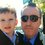# What is the sum of all positive integers less than LaTeX: $10^4$ which are relatively prime to LaTeX: $10^4?$

  1 2 3 4 5 6 7 8 9 10 11 12 13 14 15 16 17 18 19 20 21 22 23 24 25 26 27 28 def num_factors(n, p, rpt): results = [] for i in range(1, int(n**0.5) + 1): if n % i == 0: if n == p: rpt.append(i) rpt.append(int(n/i)) results.append(i) results.append(int(n/i)) if n == p: rpt = sorted(set(rpt)) rpt = rpt[1:] results = sorted(set(results)) results = results[1:] k = set(results).intersection(set(rpt)) # print k if len(k) == 0: numbers.append(n) return numbers ################################################################# p = 10000 r_p_to = [] # relatively prime to numbers = [] for j in range (p, 0, -1): l = num_factors(j, p, r_p_to) print sorted(l) print 'Number of positive integers relatively prime to %d: %d' %( p, len(l)) print 'Sum of positive integers relatively prime to %d: %d' %( p, sum(l)) 

[1, 3, 7, 9, 11, 13, 17, ......................................................, 9977, 9979, 9981, 9983, 9987, 9989, 9991, 9993, 9997, 9999]

Number of positive integers relatively prime to 10000: 4000 Sum of positive integers relatively prime to 10000: 20000000Note by Michael Fitzgerald
3 years, 5 months ago

This discussion board is a place to discuss our Daily Challenges and the math and science related to those challenges. Explanations are more than just a solution — they should explain the steps and thinking strategies that you used to obtain the solution. Comments should further the discussion of math and science.

When posting on Brilliant:

• Use the emojis to react to an explanation, whether you're congratulating a job well done , or just really confused .
• Ask specific questions about the challenge or the steps in somebody's explanation. Well-posed questions can add a lot to the discussion, but posting "I don't understand!" doesn't help anyone.
• Try to contribute something new to the discussion, whether it is an extension, generalization or other idea related to the challenge.

MarkdownAppears as
*italics* or _italics_ italics
**bold** or __bold__ bold
- bulleted- list
• bulleted
• list
1. numbered2. list
1. numbered
2. list
Note: you must add a full line of space before and after lists for them to show up correctly
paragraph 1paragraph 2

paragraph 1

paragraph 2

[example link](https://brilliant.org)example link
> This is a quote
This is a quote
    # I indented these lines
# 4 spaces, and now they show
# up as a code block.

print "hello world"
# I indented these lines
# 4 spaces, and now they show
# up as a code block.

print "hello world"
MathAppears as
Remember to wrap math in $$ ... $$ or $ ... $ to ensure proper formatting.
2 \times 3 $2 \times 3$
2^{34} $2^{34}$
a_{i-1} $a_{i-1}$
\frac{2}{3} $\frac{2}{3}$
\sqrt{2} $\sqrt{2}$
\sum_{i=1}^3 $\sum_{i=1}^3$
\sin \theta $\sin \theta$
\boxed{123} $\boxed{123}$

Sort by:

Are you testing features out or something?

- 3 years, 2 months ago